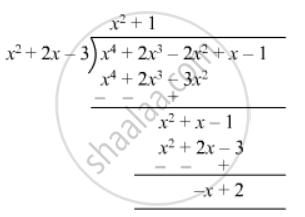Advertisement Remove all ads

# What Must Be Added to X4 + 2x3 − 2x2 + X − 1 , So that the Resulting Polynomial is Exactly Divisible by X2 + 2x − 3? - Mathematics

Sum

What must be added to x4 + 2x3 − 2x2 + x − 1 , so that the resulting polynomial is exactly divisible by x2 + 2x − 3?

Advertisement Remove all ads

#### SolutionThus, (x -  2) should be added to ($x^4 + 2 x^3 - 2 x^2 + x - 1$ ) to make the resulting polynomial exactly divisible by $(x^2 + 2x - 3)$

Is there an error in this question or solution?
Advertisement Remove all ads

#### APPEARS IN

RD Sharma Class 8 Maths
Chapter 8 Division of Algebraic Expressions
Exercise 8.4 | Q 25 | Page 12
Advertisement Remove all ads

#### Video TutorialsVIEW ALL 

Advertisement Remove all ads
Share
Notifications

View all notifications

Forgot password?
Course